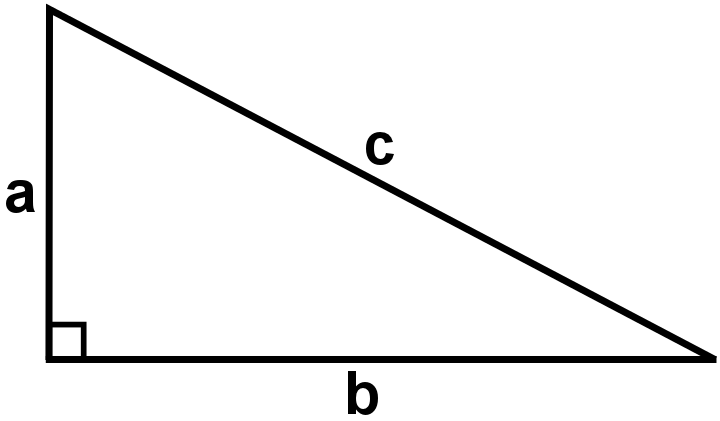#### You may also like### Diophantine N-tuples

Can you explain why a sequence of operations always gives you perfect squares?### DOTS Division

Take any pair of two digit numbers x=ab and y=cd where, without loss of generality, ab > cd . Form two 4 digit numbers r=abcd and s=cdab and calculate: {r^2 - s^2} /{x^2 - y^2}.### Sixational

The nth term of a sequence is given by the formula n^3 + 11n . Find the first four terms of the sequence given by this formula and the first term of the sequence which is bigger than one million. Prove that all terms of the sequence are divisible by 6.

# Pythagoras Perimeters

##### Age 14 to 16Challenge Level

Pythagoras Perimeters printable worksheet - proof sorting
Pythagoras Perimeters printable worksheet - whole problemIf this right-angled triangle has a perimeter of $12$ units, it is possible to show that the area is $36-6c$ square units.

Can you find a way to prove it?
Once you've had a chance to think about it, click below to see a possible way to solve the problem, where the steps have been muddled up.
Can you put them in the correct order?

a) Squaring both sides: $a^2+2ab+b^2 = 144-24c+c^2$

b) So Area of the triangle $=36-6c$

c) $a+b=12-c$

d) So $2ab=144-24c$

e) Area of the triangle $= \frac{ab}{2}$

f) By Pythagoras' Theorem, $a^2+b^2=c^2$

g) $a+b+c=12$

h) Dividing by $2$: $ab=72-12c$

Printable Version

Can you adapt your method, or the method above, to prove that when the perimeter is $30$ units, the area is $225 - 15c$ square units?

Extension
Can you find a general expression for the area of a right angled triangle with hypotenuse $c$ and perimeter $p$?

With thanks to Don Steward, whose ideas formed the basis of this problem.